﻿ LED光源电光效率测量实验研究

# LED光源电光效率测量实验研究Experimental Research of Electro-Optic Efficiency of LED Light Sources

Abstract: The luminous flux of red, green and blue light source was measured with integrating sphere as the light receiver, and then the electro-optic conversion efficiency was calculated. The electro-optic conversion efficiency of LED decreases with increasing of current. The factors affecting the conver-sion efficiency of LED light sources are analyzed.

1. 引言

2. 积分球测试原理

${E}_{M}={E}_{1}+\frac{\rho }{1-\rho }\cdot \frac{\Phi }{4\pi {r}^{2}}$ (1)

${E}_{M}=\frac{\rho }{1-\rho }\cdot \frac{\Phi }{4\pi {r}^{2}}$ (2)

${E}_{S}=\frac{\rho }{1-\rho }\cdot \frac{{\Phi }_{S}}{4\pi {r}^{2}}$ (3)

${E}_{c}=\frac{\rho }{1-\rho }\cdot \frac{{\Phi }_{c}}{4\pi {r}^{2}}$ (4)

${\Phi }_{c}=\frac{{E}_{c}}{{E}_{s}}\cdot {\Phi }_{s}$ (5)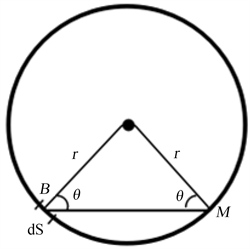Figure 1. Schematic diagram of integrating sphere luminous flux principle

3. 实验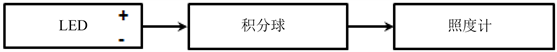Figure 2. Experimental diagram of LED light flux measurement

4. 数据分析

${\eta }_{\text{l}}=\frac{{\Phi }_{\text{l}}}{U\cdot {\text{I}}_{\text{l}}}$ (6)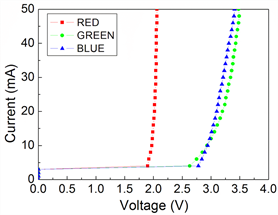Figure 3. Volt versus current curve of different types of LED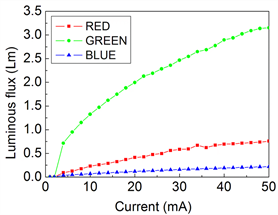Figure 4. Relation curve of luminous flux versus current of different types of LED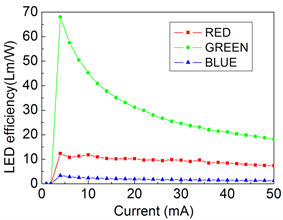Figure 5. Relationship between electro optic efficiency and current of different types of LED

5. 结论

2019年海南省基础与应用基础研究计划(自然科学领域)高层次人才项目(2019RC190, 2019RC192)；海南省自然科学基金(2018CXTD336, 618QN241)，海南省高等学校科学研究项目(Hnky2020ZD-12, Hnky2020-24)资助。

NOTES

*通讯作者。

 Dupuis, R.D. and Krames, M.R. (2008) History, Development and Applications of High-Brightness Visible Light-Emitting Diodes. Journal of Lightwave Technology, 26, 1154-1171.
https://doi.org/10.1109/JLT.2008.923628

 Niu, N., Wang, H., Liu, J., et al. (2007) Enhanced luminescence of InGaN/GaN Multiple Quantum Wells by Strain Reduction. Solid State Electronics, 51, 860-864.
https://doi.org/10.1016/j.sse.2007.04.007

 张晶晶, 张涛, 郑奇, 孟杰. 大功率LED在线性及PWM调光下的电光转换效率及热分析[J]. 光电子•激光, 2013, 24(1): 50-55.

 陈风, 袁银麟, 郑小兵. 发光二极管在光学辐射测量中的应用及进展[J]. 激光与光电子学进展, 2008(4): 57-62.

 李伟铭, 柳挺, 黄河山, 周钢, 吴杜雄. 半导体照明产品的光通量测量方法研究[J]. 电子测试, 2019(2): 73-75..

 高金环, 张磊, 高兆丰, 黄杰, 徐立生. LED光源寿命评估方法探讨[J]. 半导体技术, 2013, 38(12): 946-948.

 刘向远, 张穗萌, 徐文清, 范传宇, 王锦. 分光光谱法与光度法测量灯具光通量自吸收效应补偿的差异[J]. 激光与光电子学进展, 2016, 53(10): 132-138.

 Gorrotxategi, P., Consonni, M. and Gasse, A. (2015) Optical Efficiency Characterization of LED Phos-phors Using a Double Integrating Sphere System. Journal of Solid State Lighting, 2, 1-14.
https://doi.org/10.1186/s40539-014-0020-7

 徐玉珍, 林维明. 一种简化变量的新型LED光电热模型[J]. 光学学报, 2013, 33(5): 230-236.

 宁磊, 史永胜, 史耀华, 陈阳阳. LED封装结构对出光率的影响[J]. 液晶与显示, 2010, 25(6): 822-825.

 金毓铨, 陶有迁, 王因生, 韩钧, 施传贵. 关于半导体器件热特性表征和控制技术的研究[J]. 固体电子学研究与进展, 2011, 31(1): 53-55.

 崔德胜, 郭伟玲, 崔碧峰, 闫薇薇, 刘莹. 注入电流对GaN基LED发光特性的影响[J]. 光电子•激光, 2011, 22(9): 1309-1312.

Top